## Elementary Row Operations

In linear algebra, there are 3 elementary row operations. The same operations can also be used for column (simply by changing the word “row” into “column”). The elementary row operations can be applied to a rectangular matrix size m by n.

1. Interchanging two rows of the matrix. Notation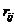means to interchange row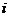and row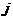.
2. Multiplying a row of the matrix by a scalar. Notation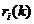means to multiply rowby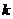,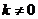.
3. Add a scalar multiple of a row to another row. Notation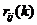means to addtimes the rowto row,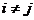.

Applying the three elementary row operations to a matrix will produce row equivalent matrix. When we view a matrix as augmented matrix of a linear system, the three elementary row operations are equivalent to interchanging two equations, multiplying an equation by a non-zero constant and adding a scalar multiple of one equation to another equation. Two linear systems are equivalent if they produce the same set of solutions. Since a matrix can be seen as a linear system, applying the 3 elementary row operations does not change the solutions of that matrix.

The 3 elementary row operations can be put into 3 elementary matrices. Elementary matrix is a matrix formed by performing a single elementary row operation on an identity matrix. Multiplying the elementary matrix to a matrix will produce the row equivalent matrix based on the corresponding elementary row operation.

Elementary Matrix Type 1: Interchanging two rows of the matrix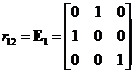,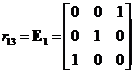,Elementary Matrix Type 2: Multiplying a row of the matrix by a scalar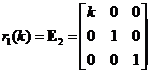,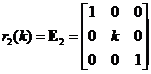,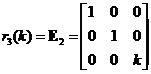Elementary Matrix Type 3: Add a scalar multiple of a row to another row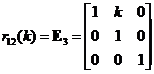,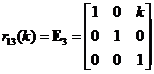,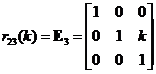,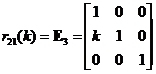,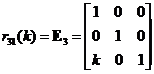,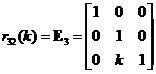Example:
Suppose we have matrix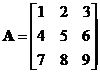Applying elementary matrix type 3 of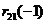produces row equivalent matrixApplying elementary matrix type 2 ofto the last result produces row equivalent matrix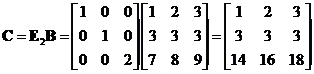Applying elementary matrix type 1 of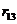to the last result produces row equivalent matrix